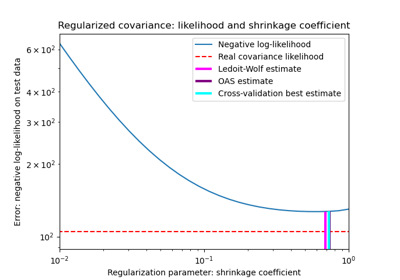# sklearn.covariance.empirical_covariance¶

sklearn.covariance.empirical_covariance(X, assume_centered=False)[source]

Computes the Maximum likelihood covariance estimator

Parameters
Xndarray, shape (n_samples, n_features)

Data from which to compute the covariance estimate

assume_centeredboolean

If True, data will not be centered before computation. Useful when working with data whose mean is almost, but not exactly zero. If False, data will be centered before computation.

Returns
covariance2D ndarray, shape (n_features, n_features)

Empirical covariance (Maximum Likelihood Estimator).

## Examples using sklearn.covariance.empirical_covariance¶